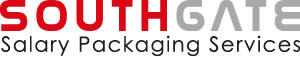RCM

Depending on your gross salary, the Recipient Contribution Method (RCM) of salary packaging a Novated Lease vehicle may improve the tax effectiveness of your salary package. The RCM is used to reduce the Taxable Value of a Novated Lease vehicle to zero (thereby eliminating the FBT liability) by contributing post tax dollars towards the payment of Novated Lease expenses, and providing a further benefit that would not be achieved without using RCM.

Under the RCM, the total lease and running costs of the vehicle are met through a combination of pre and post tax income. This requires the employee to make a post-tax contribution towards the running costs of the motor vehicle through their salary package. The post-tax contribution must be equal to the taxable value of the vehicle to fully eliminate the FBT liability, as demonstrated below using the formula for FBT calculation.

If Taxable Value equals zero, then:

 Fringe Benefits Tax (FBT) = Taxable Value x Gross Up Factor x FBT Rate = 0 x Gross Up Factor x FBT Rate = 0

Southgate arranges the payment of RCM by advising your employer’s payroll department to deduct the appropriate amount of pre and post tax income from your salary. The RCM is an attractive option for salary packaging a Novated Lease for those employees on a marginal tax income rate of less than 47% (ie. income below \$180,000). However, there will not be a detrimental impact on employees on the top marginal rate of income tax (47% including Medicare Levy).

Under the A New Tax System (Goods and Services Tax) Act 1999, ITCs cannot be claimed by an employer for any post tax contributions made by an employee towards their Novated Lease operating costs. This will reduce the ITCs available to the employer to be returned to the employee’s salary package.

Example: Calculating the RCM amount

Assumptions:

• Vehicle cost base = \$30,000 (excludes on-road costs)
• Annual kilometres = 15,000kms (20% statutory percentage)
• Annual vehicle operating costs = \$13,000
• Days available for private use = 365 days

Step 1: Calculate the Taxable Value

 Taxable Value of Vehicle = [(A x B) x C ÷ D] = [(30,000 x 0.20) x 365/365] = \$6,000

As established above, the taxable value is equal to the recipient contribution (post-tax); therefore in this example, the recipient contribution will need to be \$6,000 to reduce the FBT liability to zero.

Step 2: Calculate the Pre and Post Tax Amounts

 Total Running Cost = Pre Tax Salary Deduction + Recipient Contribution (post-tax) Therefore, Pre Tax Salary Deduction = Total Operating Cost – Recipient Contribution (post-tax) = \$13,000 – \$6,000 = \$7,000

As the total lease and running costs of the vehicle are equal to \$13,000 with the recipient contribution (post tax) amount needing to be \$6,000 to eliminate the FBT liability, the pre-tax salary deduction must be \$7,000. This combination of pre and post tax reduces the taxable value of the vehicle to zero, and hence the FBT to zero.